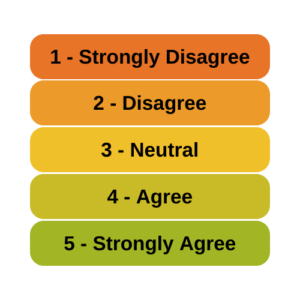# OptinMon 02 - Binary, Ordinal, and Multinomial Logistic Regression...

### Can Likert Scale Data ever be Continuous?

January 19th, 2023 by

A very common question is whether it is legitimate to use Likert scale data in parametric statistical procedures thatrequire interval data, such as Linear Regression, ANOVA, and Factor Analysis.

A typical Likert scale item has 5 to 11 points that indicate the degree of something. For example, it could measure agreement with a statement, such as 1=Strongly Disagree to 5=Strongly Agree. It can be a 1 to 5 scale, 0 to 10, etc. (more…)

### When Linear Models Don’t Fit Your Data, Now What?

June 20th, 2022 by

When your dependent variable is not continuous, unbounded, and measured onan interval or ratio scale, linear models don’t fit. The data just will not meet the assumptions of linear models. But there’s good news, other models exist for many types of dependent variables.

Today I’m going to go into more detail about 6 common types of dependent variables that are either discrete, bounded, or measured on a nominal or ordinal scale and the tests that work for them instead. Some are all of these.

### Member Training: Explaining Logistic Regression Results to Non-Researchers

August 1st, 2020 byInterpreting the results of logistic regression can be tricky, even for people who are familiar with performing different kinds of statistical analyses. How do we then share these results with non-researchers in a way that makes sense?

### Five Ways to Analyze Ordinal Variables (Some Better than Others)

April 14th, 2020 by

There are not a lot of statistical methods designed just to analyze ordinal variables.But that doesn’t mean that you’re stuck with few options.  There are more than you’d think.

Some are better than others, but it depends on the situation and research questions.

Here are five options when your dependent variable is ordinal.
(more…)

### How to Decide Between Multinomial and Ordinal Logistic Regression Models

March 11th, 2019 by

A great tool to have in your statistical tool belt is logistic regression.

It comes in many varieties and many of us are familiar with the variety for binary outcomes.

But multinomial and ordinal varieties of logistic regression are also incredibly useful and worth knowing.

They can be tricky to decide between in practice, however.  In some — but not all — situations you (more…)

### How to Understand a Risk Ratio of Less than 1

December 26th, 2018 by

When a model has a binary outcome, one common effect size is a risk ratio. As a reminder, a risk ratio is simply a ratio of two probabilities. (The risk ratio is also called relative risk.)

Risk ratios are a bit trickier to interpret when they are less than one.

A predictor variable with a risk ratio of less than one is often labeled a “protective factor” (at least in Epidemiology). This can be confusing because in our typical understanding of those terms, it makes no sense that a risk be protective.